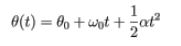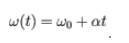# Problem: Rotational motion with a constant nonzero acceleration is not uncommon in the world around us. For instance, many machines have spinning parts. When the machine is turned on or off, the spinning parts tend to change the rate of their rotation with virtually constant angular acceleration. Many introductory problems in rotational kinematics involve motion of a particle with constant, nonzero angular acceleration. The kinematic equations for such motion can be written as:andIn answering the following questions, assume that the angular acceleration is constant and nonzero: True or FalseA) The quantity represented by θ is a function of time (i.e., is not constant)B) The quantity represented by θ0 is a function of time (i.e., is not constant).

###### FREE Expert Solution

A)

θ is the angular position measured in radians. The angular position changes with time.

82% (196 ratings)###### Problem Details

Rotational motion with a constant nonzero acceleration is not uncommon in the world around us. For instance, many machines have spinning parts. When the machine is turned on or off, the spinning parts tend to change the rate of their rotation with virtually constant angular acceleration. Many introductory problems in rotational kinematics involve motion of a particle with constant, nonzero angular acceleration. The kinematic equations for such motion can be written as:andIn answering the following questions, assume that the angular acceleration is constant and nonzero:

True or False

A) The quantity represented by θ is a function of time (i.e., is not constant)

B) The quantity represented by θ0 is a function of time (i.e., is not constant).